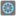• # last test case fails (-45)*-45 = 2045

## Question related to missionEven the Last

```def checkio(array: list) -> int:
"""
sums even-indexes elements and multiply at the last
"""
if not array:#check array is empty
return 0
else:
total = 0 # variable to store total
last = len(array) - 1
for i in range(0,array[last]):# loop through to last index of list
if i%2==0:# check if index number is even
total += i#add index to total

#These "asserts" using only for self-checking and not necessary for auto-testing
if __name__ == '__main__':
print('Example:')
print(checkio([0, 1, 2, 3, 4, 5]))

assert checkio([0, 1, 2, 3, 4, 5]) == 30, "(0+2+4)*5=30"
assert checkio([1, 3, 5]) == 30, "(1+5)*5=30"
assert checkio() == 36, "(6)*6=36"
assert checkio([]) == 0, "An empty array = 0"
print("Coding complete? Click 'Check' to review your tests and earn cool rewards!")
```7